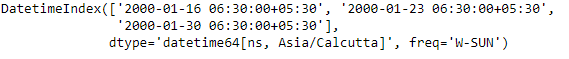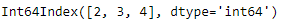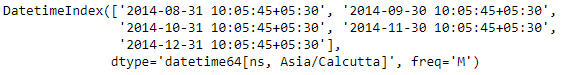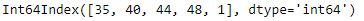# Python | Pandas DatetimeIndex.week

• Last Updated : 24 Dec, 2018

Python is a great language for doing data analysis, primarily because of the fantastic ecosystem of data-centric python packages. Pandas is one of those packages and makes importing and analyzing data much easier.

Pandas` DatetimeIndex.week` attribute outputs the ordinal value of the week for each entries of the DatetimeIndex object. It is similar to the `DatetimeIndex.week` attribute.

Syntax: DatetimeIndex.week

Return: Index object

Example #1: Use `DatetimeIndex.week` attribute to find the ordinal value of the week for each entries in the DatetimeIndex object.

 `# importing pandas as pd``import` `pandas as pd`` ` `# Create the DatetimeIndex``# Here 'W' represents Weekly frequency``didx ``=` `pd.DatetimeIndex(start ``=``'2000-01-10 06:30'``, freq ``=``'W'``,``                            ``periods ``=` `3``, tz ``=``'Asia/Calcutta'``)`` ` `# Print the DatetimeIndex``print``(didx)`

Output :Now we want to find ordinal value of the week for each entries in the DatetimeIndex object.

 `# find the ordinal value of the week``# for each entries present in the object``didx.week `

Output :As we can see in the output, the function has returned an Index object containing the ordinal values of the weeks present in each entry of the DatetimeIndex object.

Example #2: Use `DatetimeIndex.week` attribute to find the ordinal value of the week for each entries in the DatetimeIndex object.

 `# importing pandas as pd``import` `pandas as pd`` ` `# Create the DatetimeIndex``# Here 'M' represents monthly frequency``didx ``=` `pd.DatetimeIndex(start ``=``'2014-08-01 10:05:45'``, freq ``=``'M'``, ``                               ``periods ``=` `5``, tz ``=``'Asia/Calcutta'``)`` ` `# Print the DatetimeIndex``print``(didx)`

Output :Now we want to find ordinal value of the week for each entries in the DatetimeIndex object.

 `# find the ordinal value of the week ``# for each entries present in the object``didx.week`

Output :As we can see in the output, the function has returned an Index object containing the ordinal values of the weeks present in each entry of the DatetimeIndex object.

My Personal Notes arrow_drop_up